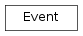# mvpa2.misc.support.Event¶class `mvpa2.misc.support.``Event`(**kwargs)

Simple class to define properties of an event.

The class is basically a dictionary. Any properties can be passed as keyword arguments to the constructor, e.g.:

```>>> ev = Event(onset=12, duration=2.45)
```

Conventions for keys:

`onset`
The onset of the event in some unit.
`duration`
The duration of the event in the same unit as `onset`.
`label`
E.g. the condition this event is part of.
`chunk`
Group this event is part of (if any), e.g. experimental run.
`features`
Any amount of additional features of the event. This might include things like physiological measures, stimulus intensity. Must be a mutable sequence (e.g. list), if present.

Methods

 `as_descrete_time`(dt[, storeoffset, offsetattr]) Convert `onset` and `duration` information into descrete timepoints. `clear`(() -> None.  Remove all items from D.) `copy`(() -> a shallow copy of D) `fromkeys`(...) v defaults to None. `get`((k[,d]) -> D[k] if k in D, ...) `has_key`((k) -> True if D has a key k, else False) `items`(() -> list of D’s (key, value) pairs, ...) `iteritems`(() -> an iterator over the (key, ...) `iterkeys`(() -> an iterator over the keys of D) `itervalues`(...) `keys`(() -> list of D’s keys) `pop`((k[,d]) -> v, ...) If key is not found, d is returned if given, otherwise KeyError is raised `popitem`(() -> (k, v), ...) 2-tuple; but raise KeyError if D is empty. `setdefault`((k[,d]) -> D.get(k,d), ...) `update`(([E, ...) If E present and has a .keys() method, does: for k in E: D[k] = E[k] `values`(() -> list of D’s values) `viewitems`(...) `viewkeys`(...) `viewvalues`(...)
Parameters: **kwargs : dict All keys to describe the Event to initialize its dict.

Methods

 `as_descrete_time`(dt[, storeoffset, offsetattr]) Convert `onset` and `duration` information into descrete timepoints. `clear`(() -> None.  Remove all items from D.) `copy`(() -> a shallow copy of D) `fromkeys`(...) v defaults to None. `get`((k[,d]) -> D[k] if k in D, ...) `has_key`((k) -> True if D has a key k, else False) `items`(() -> list of D’s (key, value) pairs, ...) `iteritems`(() -> an iterator over the (key, ...) `iterkeys`(() -> an iterator over the keys of D) `itervalues`(...) `keys`(() -> list of D’s keys) `pop`((k[,d]) -> v, ...) If key is not found, d is returned if given, otherwise KeyError is raised `popitem`(() -> (k, v), ...) 2-tuple; but raise KeyError if D is empty. `setdefault`((k[,d]) -> D.get(k,d), ...) `update`(([E, ...) If E present and has a .keys() method, does: for k in E: D[k] = E[k] `values`(() -> list of D’s values) `viewitems`(...) `viewkeys`(...) `viewvalues`(...)
`as_descrete_time`(dt, storeoffset=False, offsetattr='offset')

Convert `onset` and `duration` information into descrete timepoints.

Parameters: dt : float Temporal distance between two timepoints in the same unit as `onset` and `duration`. storeoffset : bool If True, the temporal offset between original `onset` and descretized onset is stored as an additional item. offsetattr : str The name of the attribute that is used to store the computed offset in case the `storeoffset` is enabled. A copy of the original `Event` with `onset` and optionally `duration` : replaced by their corresponding descrete timepoint. The new onset will : correspond to the timepoint just before or exactly at the original : onset. The new duration will be the number of timepoints covering the : event from the computed onset timepoint till the timepoint exactly at : the end, or just after the event. : Note again, that the new values are expressed as #timepoint and not : in their original unit! :